# Effect of Tube Number on Critical Heat Flux and Thermal Performance in Linear Fresnel Collector Based on Direct Steam Generation

Effect of Tube Number on Critical Heat Flux and Thermal Performance in Linear Fresnel Collector Based on Direct Steam Generation
Department of Mechanical Engineering, Central Tehran Branch, Islamic Azad University, Tehran, Iran

Department of Mechanical Engineering, Tafresh University, Tafresh, Iran

Corresponding Author Email:
arashlavasani@iauctb.ac.ir
Page:
223-230
|
DOI:
https://doi.org/10.18280/ijht.380124
7 September 2019
|
Accepted:
25 January 2020
|
Published:
31 March 2020
| Citation

OPEN ACCESS

Abstract:

In this paper heat transfer and critical heat flux is investigated in the Linear Fresnel Collector. At first, the balance of energy in the absorber was used for the modeling of direct steam generation in the Fresnel solar plant. In the following, thermal analysis has been executed in three different mass flow rates and single and dual tubes. Eventually Critical Heat Flux and distribution of vapor quality are studied from the start of the fluid flow until critical heat flux. How to determine the critical heat flux is important so that the amount of Critical Heat Flux is obtained from the comparison of inlet heat flux and vapor quality with data in Look up table. Noteworthy, in this study, water is picked out as heat transfer fluid because considered system is Direct Steam Generation. From the analysis, the temperature of the internal tube surface cut down on in the amount of 1.6 percent in mode of double tubes than single tube. Also, critical heat flux’s length and vapor quality respectively increased in the amount of 25.2 and 9 percent. However, the point of critical heat flux has been postponed with reducing of fluid flow rate and increasing of tube numbers.

Keywords:

analysis of heat transfer, linear Fresnel concentrator, direct steam generation, critical heat flux

1. Introduction

Study about solar power plants and parameters is important so that, it causes increase in performance of the system. Solar power plants have been built for absorbing and receiving of solar energy and converting to electricity. They have been constructed two sections, including solar farm and energy exchanger for producing of electricity. In the solar power plants of the type of the linear Fresnel collectors (LFC), there are some flat mirrors with small widths that are function of sun irradiation and actually the irradiation transported from mirrors to absorber complex and eventually absorber tubes, inside of trapezoidal cavity .

Sahoo et al.  studied about fluid hydrothermal analysis in linear Fresnel concentrators for single and two phase of fluid. In 2017, Beltagy et al.  studied theoretical and experimental analysis about the increase of efficiency in linear Fresnel concentrator systems, in which the results show the increase in efficiency around 40 percent for a solar power plant with 250 KW capacity. Pakhomov et al.  presented the numerical modeling of the pattern and heat transfer in a polydisperse bubbly turbulent flow after sudden enlargement in a tube. Pino et al. studied an experimental work about validation of an optical and heat model for the LFC system with single axis tracker. In the system of solar heating converter, heat loss mechanism can increase system efficiency and decrease system cost significantly .

Rovira et al.  have studied performance of integrated solar combined cycles. These cycles use from Fresnel and parabolic through concentrators with approach of direct steam generation. The result of comparison between integrated solar combined cycles in Almeria and Las Vegas show low level of energy cost in linear Fresnel concentrator.

Antonov et al.  have done modeling the water droplet evaporation process with regard to convection, conduction and thermal radiation. They have paid to investigation of velocity and temperature profiles in the gas-water droplet system so that external gas medium temperature varied from 100 to 800 $^{\circ} \mathrm{C}$ . Bellos et al.  have done investigate about daily performance of Linear Fresnel Concentrator in energetic and exergetic. The simulation program is used to get thermal and optical efficiency diagrams and its results used in development of numerical dynamic model. As a result, the mean yearly incident angle modifier is 37% while maximum yearly exergetic is 8%. As well as, thermal efficiency increased 18.5%.

Numerical studies on Linear Fresnel Concentrator to determine absorber performance in different directs of wind done by Cagnoli et al. . Geometry of this research embraces absorber and glass tubes that space between them filled with air in 1 atm pressure. Also, from Monte Carlo method was used for analysis of absorbed heat flux. Yearly performance due to low temperature of absorber is not significant. So, direction and velocity wind to 4 meter per second on the absorber performance is insignificant. Tsekouras, Tzivanidis and Antonopoulos  have done optical and thermal investigation on the LFC with trapezoidal cavity receiver. In this research is shown development of ray-tracing model and sun irradiation distribution on absorber environs. Simulation results show domain of absorber heat less for inlet temperature of fluid between 150 to 375 Celsius respectively 181.2 to 986 watt per meter. Zhu et al.  did a research about linear Fresnel reflector scalable type that can acquire different temperatures and high heat efficiency. About decreasing of heat loss, Qiu et al.  did a research according to Monte-Carlo method and geometry of trapezoidal cavity. That way with recognition of 81 to 87 percent of radiation heat loss, they propose coating process for mirror and absorber with low emission capability. Abbas, Munoz and Martinez-Val  studied heat transfer and heat less for variety diameters of absorber tubes on the linear Fresnel collector. Thermal oil is used in this system as heat transfer fluid that the exergetic efficiency and fluid temperature reduce with increase inlet flux and diameter of absorber.

The present analysis is based on direct steam generation and distribution of internal surface temperature of tube, vapor quality and fluid temperature done for three mass flow and two different tube diameters.

The critical heat flux’s point is the important point in boiling section. Maybe, we will design absorber’s tube so that it works nearby in this point but there is a danger of temperature increase in this area and increase of thermal stress. In this research, critical heat flux is compared in different modes, involve number of different tubes and different mass flow rates. Heat transfer analysis is done for single-phase, sub-cooled and two-phase fluid flow sections for single and double tubes. Basic issue in this research is how to set the critical heat flux that can be used from Look up Table (LUT) which distinguishes this research from other researches. In this way, with comparing obtained results about applied heat flux with fluid and vapor quality in length of two-phase flow section and available data in table, selected heat flux as critical heat flux that correspond with quantity available in the LUT. Ultimately, in this paper has been obtained distribution of fluid temperature, surface internal temperature of tube and vapor quality and so their results are shown decrease of surface internal temperature of tube, increase of vapor quality and increase of length of critical heat flux in double tubes than single tube. Also, results are obtained for three different flow rates during which, decreasing of flow rate causes delay in occurrence of critical heat flux.

2. Simulation and Govering Equations in Fresnel Receiver

In this paper, the approach of general modeling is based on energy balance about heat element collector like other studies. This energy balance involves direct solar radiation, radiation losses from flat mirror, heat collector element, heat losses from heat collector element and input heat to heat transfer fluid that is visible in Figure 1. On the other side, heat transfer circuit of this process is observable Figure 2.

## 1.png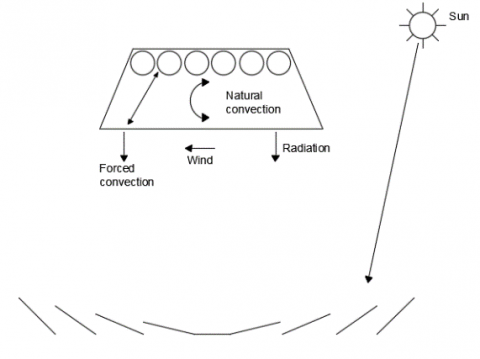Figure 1. Fresnel model 

## 2.png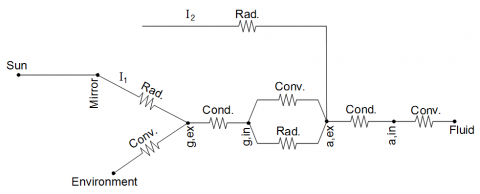Figure 2. Heat transfer circuit for Fresnel system

According to this circuit, after entering the sun heat flux to the mirror, radiation heat flux reflected from mirror to external surface of glass that its amount is $I_{1}$ . Percentage of the radiation heat flux as conduction heat flux passes from glass wall and residual radiation heat flux according to glass reflection coefficient as convection heat flux enters to the environment. The environment inside the cavity is relatively vacuum.

In the following, transitional radiation heat flux in the amount of $I_{2}$  enter to external surface of tubes inside of cavity that it was divided between tubes. In this section, according to the reflection coefficient of tube, a part of that heat flux is reflected and exchanged radiation and convection heat flux with glass internal surface. We called off the radiation heat flux between glass and tubes because the amount of this heat flux is very low. The remaining amount of heat flux transient from tubes wall as conduction heat flux and heat enter to heat transfer fluid as convection heat flux.

If number of tubes increase to N so convection heat flux from tube to glass and radiation heat flux from mirror to tube divide to number of tubes.

2.1 Radiation heat flux from mirror to glass and tube

This section expresses the equations about radiation heat flux from mirror to glass ( $\dot{q}_{g, s . r a d}$ ) and tube ( $\dot{q}_{a, s . r a d}$ ) :

$\dot{q}_{g, s . r a d}=\mathrm{I}_{s u n} \cdot \beta . \alpha_{g} . \mathrm{W}$              (1)

$\dot{q}_{a, s . r a d}=\frac{1}{N} \mathrm{I}_{s u n} \cdot \beta \cdot \gamma \cdot \alpha_{\mathrm{a}} \cdot \mathrm{W}$          (2)

In the above equations $W, N, I_{s u n}, \alpha_{a}, \alpha_{g}, \beta \text { and } \gamma$  respectively show width of total of mirrors, number of tubes, the amount of sun irradiation, absorption coefficient of tube, absorption coefficient of glass and reflection and transient coefficient of glass.

2.2 Conduction heat flux in glass and tube wall

The heat passes from tubes and glass wall in the form of conduction heat flux when radiation heat flux receives to external surface of glass and tubes. This heat flux has direct connection with glass and tube thermal conductivity coefficient . According to glass and tube material, conduction heat flux has high value when this coefficient has high value and at the result, it effects in coefficient of heat transfer for fluid and the convection heat flux from absorber to fluid increases eventually.

2.3 Convection heat flux from glass to environment

As soon as the radiation heat flux reaches to glass’s wall, the heat transfers from that and ultimately enters to cavity as convection heat flux. According to glass reflection coefficient, remaining of this flux reflects from glass and is transferred to the ambient in the form of convection heat transfer ( $\dot{q}_{g-e, \text { conv }}$ ). In the following, equations of this section are presented [2, 10]:

$\dot{q}_{g-e . c o n v}=h_{e} \cdot w_{c}\left(T_{g . e x}-T_{a m b}\right)$             (3)

The value of heat transfer coefficient is calculated the following equations.

$h_{e}=k_{e} \cdot N u_{e} / w_{c}$             (4)

$N u_{e}=0.15 R a^{\frac{1}{3}}$                (5)

$R a=\left(g \cdot \beta_{e}\left(T_{g, e x}-T_{a m b}\right) w_{c}^{3}\right) /\left(v_{e} \cdot \alpha\right)$       (6)

In the above equations $h_{e}, w_{c}, T_{g, e x}, T_{a m b}, k_{e}, N u_{e}, R a, g, \beta_{e}, v_{e} \text { and } \alpha$ respectively show heat transfer coefficient, length of bottom trapezoidal base, temperature of external surface of glass, temperature of ambient, thermal conductivity coefficient of ambient air, Nusselt number about ambient, Rayleigh number, gravitational acceleration, volumetric thermal expansion coefficient about air, kinematic viscosity about air and thermal diffusivity.

2.4 Convection heat flux from tube to fluid

After passing of conduction heat flux from tube wall, the heat in the form of convection heat transfer ( $\dot{q}_{a-f, c o n v}$ ) is transferred to fluid and increases the fluid temperature.

In the following, we express equations about convection heat flux for three section of fluid flow, including single-phase, sub-cooled and two phase.

2.5 Single-phase fluid flow section

Heat flux from tube to fluid is calculated by Eq. (7) [2, 14]:

$\dot{q}_{a-r, c o n v}=h_{f} \pi D_{a, i n}\left(T_{a, i n}-T_{m}\right)$                (7)

Parameter of $T_{m} \text { equals } T_{m}=\frac{T_{i n}+T_{o u t}}{2}$ and Nusselt number in the heat transfer coefficient equation is calculated by the following equation.

$N u_{f}=0.023\left(R e_{f}\right)^{0.8}\left(P r_{f}\right)^{0.4}$                      (8)

In the above equations $h_{f}, T_{a, i n}, T_{m}, T_{i n}, T_{o u t}, N u_{f}, R e_{f} \text { and } P r_{f}$ respectively show heat transfer coefficient of fluid, temperature of internal surface of tube, average temperature of fluid, inlet temperature of fluid, outlet temperature of fluid, Nusselt number, Reynolds number for fluid and Prandtl number for fluid.

2.6 Sub-cooled boiling section

Whenever the temperature of internal surface of the tube is reached to saturation temperature of fluid, the heat transfer fluid enters to sub-cooled section. Convection heat flux from tube to fluid is calculated as follows by Forster-Zubber :

$\dot{q}_{n b}=h_{n b} \pi D_{a, i n}\left(T_{a, i n}-T_{o u t}\right)$               (9)

The value of heat transfer coefficient is calculated by Eq. (10).

$h_{n b}=0.00122 \frac{k_{f}^{0.79} C_{p, f}^{0.45} \rho_{l}^{0.49}}{\sigma^{0.5} \mu_{f}^{0.29} h_{l v}^{0.24} \rho_{v}^{0.24}}\left(T_{a, i n}\right.$

$\left.-T_{\text {out}}\right)^{0.24} \Delta P_{\text {sat}}^{0.75}$              (10)

In the above equations $h_{n b}, \pi, D_{a, i n}, T_{a, i n}, T_{o u t}, k_{f}, C_{p, f}, \rho_{l}, \sigma, \mu_{f}, h_{l v}, \rho_{v}$ and $\Delta P_{s a t}$  respectively show heat transfer coefficient, Pi number, internal diameter of tube, external diameter of tube, thermal conductivity coefficient of fluid, specific heat of fluid in constant pressure, density of fluid in liquid mode, Stephen-Boltzmann coefficient, viscosity of fluid, enthalpy, density of fluid in vapor mode and difference of fluid pressure.

2.7 Two-phase fluid flow section

As soon as the fluid temperature reaches saturation temperature, the heat transfer fluid enters the two-phase section. The difference between this section and other sections is in the type of fluid flow and different method of operation about fluid. In this section, fluid temperature remains constant and only vapor quality changes in length of tube until critical heat flux. For this section, Shah Equation is used  as follows.

$\dot{q}_{n b}=h_{t p} \pi D_{a, i n}\left(T_{a, i n}-373.15\right)$             (11)

$h_{t p}=h_{L}\left[(1-x)^{0.8}+\frac{3.8 x^{0.76}(1-x)^{0.04}}{p r^{0.38}}\right]$            (12)

$h_{L}=0.023 R e_{l}^{0.8} P r_{l}^{0.4} \frac{k_{l}}{D_{a, i n}}$                 (13)

In the above equations $h_{t p}, \pi, D_{a, i n}, T_{a, i n}, h_{L}, x, p r, R e_{l}, P r_{l} \text { and } k_{l}$ respectively show heat transfer coefficient in two-phase mode, Pi number, internal diameter of tube, temperature of internal surface of tube, heat transfer coefficient in convection mode, vapor quality percent, ratio of saturation pressure to critical pressure, Reynolds number in liquid mode, Prandtl number in liquid mode and thermal conductivity coefficient in liquid mode.

2.8 Energy balance inside of fluid

Energy balance’s equations for inside of absorber tube change because mode of fluid convert from single-phase to sub-cooled and then to two-phase. It is noteworthy that energy balance’s equations define based on enthalpy and vapor quality in two-phase section while they are same for single-phase  and sub-cooled  sections and explain based on temperature difference of fluid in the beginning and end of fluid flow path.

2.9 Energy balance for single-phase and sub-cooled boiling sections

Since in the single-phase and sub-cooled sections, vapor quality equals to zero, specific heat and mass flow rate are two important parameters in balance of energy. Eq. (14) shows balance of energy in these sections.

$\dot{q}_{a-f, c o n v}=\frac{\dot{m} C_{p}\left(T_{\text {out}}-T_{\text {in}}\right)}{l}$      (14)

In the above equation $\dot{m}, C_{p}, T_{\text {out}}, T_{\text {in}} \text {and } l$ respectively show mass flow rate of fluid, specific heat in constant pressure, and outlet temperature of fluid, inlet temperature of fluid and length of each element from tube.

2.10 Energy balance for two-phase flow section

In this stage, fluid is entered to two-phase flow section and we see combine of liquid and vapor. As a result, important parameter in balance of energy inside of fluid is fluid enthalpy. Eq. (15) expresses this balance of energy.

$\dot{q}_{a-f, c o n v}=\frac{m_{p} h_{f g}}{l}$       (15)

In the above equation $m_{p}$ is fluid mass in each element that calculated by bottom equation.

$m_{p}=m_{\text {total}}\left(x_{\text {out}}-x_{\text {in}}\right)$              (16)

Also $m_{\text {total }}$ is total mass of flow that is calculated by $m_{\text {total}}=\rho V$ .

In the above equations $m_{p}, h_{f g}, m_{\text {total}}, x_{\text {out}}, x_{\text {in}}, \rho \text { and } V$ respectively show mass fluid in each element, enthalpy, total mass fluid, vapor quality in end of element, vapor quality in first of element, density of fluid and volume of fluid.

2.11 Convection heat flux from tube to glass

By entering the radiation heat flux to external surface of tube, some of this heat flux passed from tube wall and the remainder of it in the form of convection heat transfer $\left(\dot{q}_{a-g, c o n v}\right)$ go to glass. The following equation shows this heat flux [15, 17]:

$\dot{q}_{a-g . c o n v}=\frac{1}{N} h_{c} l\left(T_{a, e x}-T_{g, i n}\right)$             (17）

In the above equation $N, h_{s}, l, T_{a, e x} \text { and } T_{g, i n}$ respectively show number of tube, heat transfer coefficient about cavity, length of element, temperature of external surface of tube and temperature of internal surface of tube.

2.12 Balance of energy in set of absorber

Actually, the first law of thermodynamic used as a helpful tool for more problem of heat transfer . For the expression of this law, first we should specify the control volume. In this model, we can consider two control volume include control volume for glass and control volume for tube. Eqns. (18) and (19) respectively are equations about conversion of energy in glass and tube.

$\dot{q}_{g, s . r a d}+\left(N . \dot{q}_{a-g . c o n v}\right)-\dot{q}_{g-e, c o n v}=0$       (18)

$\dot{q}_{a, s, r a d}-\dot{q}_{a-f, c o n v}-\dot{q}_{a-g . c o n v}=0$            (19)

Also, two balance of energy are established for surfaces of glass and tube that come in Eqns. (20) and (21).

$\dot{q}_{g, \text {cond}}+\dot{q}_{a-g . c o n v}=0$                    (20)

$\dot{q}_{a, \text {cond}}-\dot{q}_{a-f . c o n v}=0$                   (21)

3. Solution Algorithm

Numerical solution of this equation is based on the use of one dimensional elements method under the conditions of steady state also, the iteration technique based on the Gauss-Seidel method has applied as the solution procedure and also, the look-up table (LUT) method has been used for calculating the critical heat flux (CHF). In this operation, for calculation of unknowns, at the beginning, we considered input parameters and boundary conditions in code calculations and then unknowns solved by solution of equations set embrace 12 equations and 12 unknowns based on iteration process. This stage is done for three section of fluid flow. Also, Razmmand et al.  have investigated a numerical analysis regarding the effect of nanofluids on heat transfer of the solar parabolic trough collector. In truth, they used from the CHF LUT for calculation of the CHF and length of the CHF point while the CHF LUT was used on linear Fresnel collector in this paper.

## 3.png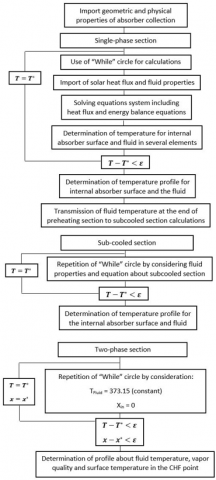Figure 3. Solution algorithm

In this research, inlet temperature of fluid considered equal to 303.15 Kelvin. Single-phase ends if temperature of internal surface of tube reaches to saturation temperature of fluid. As a result, in this time the fluid enter to sub-cooled section. In this section, fluid continues to the point that fluid temperature reached to saturation temperature. Whenever fluid temperature reaches saturation temperature, sub-cooled section ends and fluid enter to two-phase section. By entering of fluid to two-phase section, take place an important event takes place that according to it fluid temperature is constant and changes vapor quality. As the same way, temperature of internal surface of tube increases. For determining the critical heat flux or the point of end of two-phase section, is used from Look up Table . In this table, vapor quality is presented based on heat flux entered to fluid. Then, the heat flux is obtained from the analysis of comparing existing fluxes in LUT table. If heat flux is obtained from analysis seen in the LUT table, then this value is equal to critical heat flux and vapor quality about it is vapor quality equal to critical heat flux. The solution algorithm specified in Figure 3.

4. Validation

In this study, the validation is done based on Sahoo and colleagues’ research work . Sahoo did hydrothermal analysis for fluid in linear Fresnel concentrator. This analysis is for two section of fluid flow embrace single-phase and two-phase. Also, for hydrothermal analysis was used from one dimensional model in both sections. It is worth mentioning, for solution of this one dimensional model, has used from solution of energy balance equations. In Table 1, geometrical and thermal properties was shown.

The used fluid is water for validation that its result shown in Figure 4. Difference of distribution of bulk fluid temperature in the first path and in two-phase section is insignificant and only exist difference of 1.9 percent than Sahoo’s results in the start of two-phase flow. In this validation, in single-phase section exerted pressure was enhanced so that eventually it becomes equal to 1.4 bar in two-phase section.

According to this, saturation temperature of fluid increased in two-phase section. Since, used fluid is water and as we know the water after passing of sub-cooled section entered to two-phase flow, then fluid temperature is constant in this section and only vapor quality changed in length of fluid flow path. So, fluid temperature was constant and equal saturation temperature of fluid (373.15 Kelvin).

## 4.png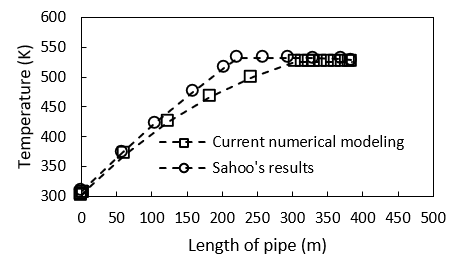Figure 4. Comparison of distribution of bulk fluid temperature in length of path in done analysis by Sahoo et al. 

Table 1. geometrical and thermal properties about set of linear Fresnel concentrator 

 Parameter Value Bottom width of cavity 500 Top width of cavity 300 Height of cavity 100 Internal diameter of tube 26.7 External diameter of tube 33.4 Length of tube 384 Fluid temperature 303 Sun irradiation 933 Environment temperature 298.15 Wind speed 0.03
5. Results

In this section, we explained the obtained results in this research. In the analysis of two modes of single tube and double tubes, distribution of fluid temperature, temperature of internal surface of tube and vapor quality obtained in three section of flow including single-phase, sub-cooled and two-phase. We consider length of tube equal 100 meters. In Table 2 and Table 3 came physical specifications of Fresnel system and thermos-physical of fluid.

Table 2. Physical properties of Fresnel system

 Parameter Value Absorbance coefficient of tube 0.96 Absorbance coefficient of glass 0.05 Environment temperature 296 Width of absorber plate 0.49 Reflex coefficient of mirror 0.93 Input temperature of fluid 303.15 Height of cavity 0.1 Big base of trapezoidal 0.6

Table 3. Thermo physical properties of pure water 

 Parameter Value Specific heat 4188 Viscosity 420 E -6 Coefficient of thermal conductivity 660 E -3 Density 979.43

## 5.pngFigure 5. Distribution of fluid temperature in length of path until critical heat flux in two mode

The important result of this analysis is comparison of critical heat flux’s amount at two modes that it has shown in fluid temperature, temperature of internal surface and vapor quality’s diagrams. In Figures 5, 6 and 7, the results of fluid temperature, internal surface temperature of tube, and vapor quality, respectively are visible for the LFC with single and double tube with tube diameter respectively 28.4 (mm) and 16.3 (mm) for amount of mass flow rate equal 0.23 (kg/s).

## 6.png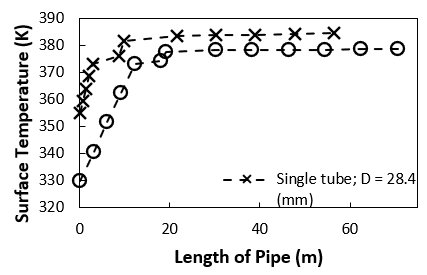Figure 6. Distribution of temperature of internal surface in length of path until critical heat flux in two mode

## 7.png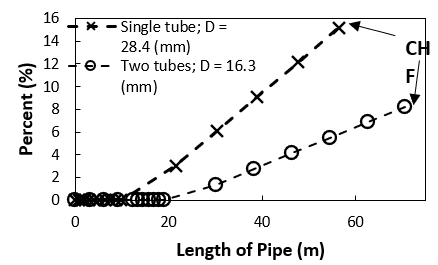Figure 7. Distribution of vapor quality in length of path until critical heat flux in two mode

## 8.png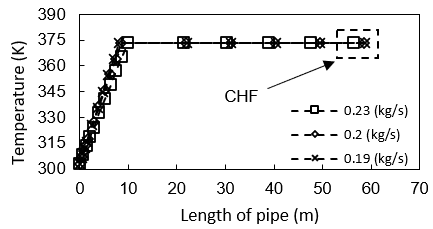Figure 8. Distribution of fluid temperature in mode of single tube for three mass flow rates

As observed in the Figures 5 to 7, we see two important events in the LFC in two tubes mode than single tube mode. According to these Figures, heat transfer fluid passes the tube path by some delay in mode of double tubes than single tube so that we see an increase of 25.2 percent in the incidence of critical heat flux. In other word, critical heat flux occurs in length of 56.39 meters of absorber tube in complex of single tube. However, in complex of double tubes, we see incidence of critical heat flux in a length of 70.62 meter. Decrease of internal surface temperature of tube and increase of vapor quality is second important incident in the complex of double tubes than the complex of single tube. So, the internal surface temperature of tube and vapor quality in point of critical heat flux in complex of double tubes respectively is reduced by 1.6 percent and increases by 9 percent than complex of single tube.

In the second mode, we obtain the results separately in the complex of double and single tubes for three different mass flow rates of 0.23, 0.2 and 0.19. The results are visible in Figures 8 to 10 for the complex of single tube.

## 9.pngFigure 9. Distribution of internal surface temperature of tube in mode of single tube for three mass flow rates

## 10.pngFigure 10. Distribution of vapor quality in mode of single tube for three mass flow rates

As it can be seen in the above figures, in point of critical heat flux we see delay in incidence of critical heat flux by decrease of mass flow rate of fluid so that there is an increase of 3.8 percent of length of critical heat flux in mass flow rate equal 0.2 than 0.23 and so there is an increase 4.2 percent of length of critical heat flux in mass flow rate equal 0.19 than 0.23.

So, up analysis done for complex of double tubes that its results come in Figures 11 to 13.

## 11.png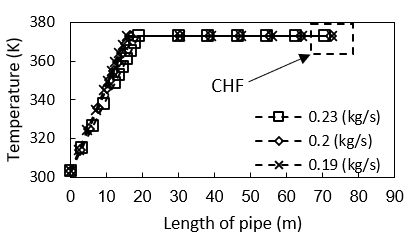Figure 11. Distribution of fluid temperature in mode of double tubes for three mass flow rates

## 12.pngFigure 12. Distribution of internal surface temperature of tube in mode of double tubes for three mass flow rates

## 13.png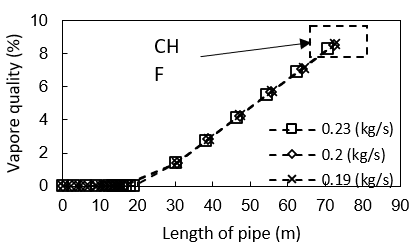Figure 13. Distribution of vapor quality in mode of double tubes for three mass flow rates

In the above tableures we observe a delay in the incidence of critical heat flux so that there is an increase of 2.2 percent of length of critical heat flux in mass flow rate equals 0.2 than 0.23 and so there is an increase of 3 percent of length of critical heat flux in mass flow rate equal 0.19 than 0.23.

6. Conclusion

In this research, the heat transfer analysis is as regards a model of the linear Fresnel collector in two modes of single and double tubes for three sections of single-phase, sub-cooled and two-phase.

The distribution of fluid temperature, internal surface temperature of tube and vapor quality is studied in this research. With due to attention to the type of system that is considered from type of direct steam generation, the water is used as a heat transfer fluid. So, geometry of cavity is considered trapezoidal type.

In this study was used from performing code calculation and in every section of fluid regime, iterative method based on Gauss-Seidel approach was entered by “While” construct in code calculation. The scrutinizing of critical heat flux (CHF) in nanofluids that is the most important part of modeling is obtained from the Look up Table (LUT) method  and this approach has been distinguished current research rather than other works. In this table, the vapor quality is presented in the order of the heat flux entered into the fluid. The obtained results of convective heat flux in two-phase section of fluid flow in each element were contrasted with Look up Table’s heat fluxes. The location of CHF is shown when the acquired convective heat flux been larger than corresponding element in Look up Table’s data. In the following, distribution of internal surface temperature of tube, distribution of fluid temperature, and distribution of vapor quality are investigated.

The most important target of this research is the comparison of the incidence of critical heat flux in different modes. First, comparison is done for complexes of single tube and double tubes by mass flow rate of fluid equals 0.23 that in complex of double tubes there is an increase of 21.6 percent in length of the incidence of critical heat flux, a decrease of 1.6 in the distribution of internal surface temperature of tube and a decrease of 9 percent of vapor quality than complex of single tube. Consequently, the analysis for three different of mass flow rate including 0.23, 0.2 and 0.19 in complexes of single tube and double tubes are done separately. As a result, there is an increase of 3.8 percent in length of critical heat flux in mass flow rate equals 0.2 than 0.23 and so there is an increase of 4.2 percent in length of critical heat flux in mass flow rate equals 0.19 than 0.23 for the complex of single tube. So, for the complex of double tubes, the increase of 2.2 percent in length of critical heat flux in mass flow rate equals 0.2 than 0.23 and an increase of 3 percent in length of critical heat in mass flow rate equals 0.19 than 0.23 can be seen.

Nomenclature
 $\mathcal{C}_{p}$ Specific heat at constant pressure, J/kg.K D Diameter, m h Convection heat transfer coefficient, W/m2.K $h_{L}$ Convection heat transfer coefficient in one-phase, W/m2.K $h_{l v}$ Latent heat of fusion, J/kg I Sun irradiation, W/m k Thermal conductivity, W/m.K $l$ Element length of pipe, m m Mass, kg $\dot{m}$ Mass flow rate, kg/s N Number of tubes $N u$ Nusselt number p Pressure, N/m2 $p r$ Prandtl number $p r$ Rate between saturate pressure and critical pressure q Heat transfer rate, W $\dot{q}$ Heat transfer rate per unit length, W/m $R a$ Rayleigh number Re Reynolds number T Temperature, K V Volume, m3 W Width of total of mirrors, m w Length of bottom trapezoidal base, m x Vapor quality, percent (%) Greek symbols α Absorption coefficient, percent (%); thermal diffusivity, m2/s β Coefficient of reflection, percent (%); volumetric thermal expansion coefficient, K-1 γ Transient coefficient, (%) $\mu$ Viscosity, kg/s.m ν Kinematic viscosity, m2/s $\pi$ Pi number, 3.14 $\rho$ Mass density, kg/m3 $\sigma$ Stefan-Boltzmann constant, W/m2.K4 $\Delta$ Difference Subscripts $a$ Absorber c Cavity cond Conduction conv Convection e Environment ex External f Fluid g Glass in Internal (for temperature), Inlet (for fluid flow) l Liquid m Middle nb Nucleate boiling out Outlet rad Radiation s Sun Sat Saturate tp Two-Phase v Vapor
References

 Gabriel, M., Dersch, J., Platzer, W., Eck, M., Haberle, A. (2012). Comparison of linear fresnel and parabolic trough collector power plants. Solar Energy, 86(1): 1-12. https://doi.org/10.1016/j.solener.2011.06.020

 Sahoo, S.S., Singh, S., Banerjee, R. (2013). Steady state hydrothermal analysis of the absorber tubes used in Linear Fresnel Reflector solar thermal system. Solar Energy, 87: 84-95. https://doi.org/10.1016/j.solener.2012.10.002

 Beltagy, H., Semmar, D., Lehaut, C., Said, N. (2017). Theoretical and experimental performance analysis of a Fresnel type solar concentrator. Renewable Energy, 101: 782-793. https://doi.org/10.1016/j.renene.2016.09.038

 Pakhomov, M.A., Terekhov, V.I. (2018). Modeling the effect of bubbles on a pattern and heat transfer in a turbulent polydisperse upward two-phase flow after sudden enlargement in a tube. High Temperature, 56(1): 52-60. https://doi.org/10.1134/S0018151X17060153

 Pino, F.J., Caro, R., Rosa, F., Guerra, J. (2013). Experimental validation of an optical and thermal model of a linear Fresnel collector system. Applied Thermal Engineering, 50(2): 1463-1471. https://doi.org/10.1016/j.applthermaleng.2011.12.020

 Roviera, A., Barbero, R., Montes, M.J., Abbas, R., Varela, F. (2016). Analysis and comparison of Integrated Solar Combined Cycles using parabolic troughs and linear Fresnel reflectors as concentrating systems. Applied Energy, 162: 990-1000. https://doi.org/10.1016/j.apenergy.2015.11.001

 Antonov, D.V., Vysokomonaya, O.V., Kuznetsov, G.V., Piskuniv, M.V. (2018). Modeling the water droplet evaporation process with regard to convection, conduction and thermal radiation. Journal of Engineering Thermophysics, 27: 145-154. https://doi.org/10.1134/S1810232818020029

 Bellos, E., Tzivanidis, B., Papadopoulos, A. (2018). Daily, monthly and yearly performance of a linear Fresnel reflector. Solar Energy, 173: 517-529. https://doi.org/10.1016/j.solener.2018.08.008

 Cagnoli, M., Massei, D., Procopio, M., Russo, V., Savoldi, L., Zanino, R. (2018). Analysis of the performance of linear Fresnel collector: Encapsulated vs. evacuated tubed. Solar Energy, 164: 119-138. https://doi.org/10.1016/j.solener.2018.02.037

 Tsekouras, P., Tzivanidis, C., Antonopoulos, K. (2018). Optical and thermal investigation of a linear Fresnel collector with trapezoidal. Applied Thermal Engineering, 135: 379-388. https://doi.org/10.1016/j.applthermaleng.2018.02.082

 Zhu, Y.Q., Shi, J.F., Li, Y.J., Wang, L.L., Huang, Q.Z., Xu, G. (2017). Design and thermal performance of a scalable linear Fresnel reflector solar system, Energy Conversion and Management, 146: 174-181. https://doi.org/10.1016/j.enconman.2017.05.031

 Yu, Q., He, Y.L., Wu, M., Zheng, Z.J. (2016). A comprehensive model for optical and thermal characterization of a linear Fresnel solar reflector with a trapezoidal cavity receiver. Renewable Energy, 97: 129-144. https://doi.org/10.1016/j.renene.2016.05.065

 Abbas, A., Munoz, J., Martinez-Val, J.M. (2012). Steady-state thermal analysis of an innovative receiver for linear Fresnel reflectors. Applied Energy, 92: 503-515. https://doi.org/10.1016/j.apenergy.2011.11.070

 Incropera, F.P., Dewitt, D.P. (2017). Fundamentals of Heat and Mass Transfer. Seventh ed. New York, USA: John Wiley & Sons Inc.

 Sarma, P.K., Srinivas, V., Sharma, K.V., Subrahmanyam, T., Kakac, S. (2008). A correlation to predict heat transfer coefficient in nucleate boiling on cylindrical heating elements. International Journal of Thermal Sciences, 47(3): 347-354. https://doi.org/10.1016/j.ijthermalsci.2007.03.003

 Shah, M.M. (1979). A general correlation for heat transfer during film condensation inside pipe. International Journal of Heat and Mass Transfer, 22(4): 547-556. https://doi.org/10.1016/0017-9310(79)90058-9

 Pye, J.D. (2008). System Modelling of the Compact Linear Fresnel Reflector, 2008, PhD diss., University of New South Wales.

 Razmmand, F., Mehdipour, R. (2019). Studying thermal stresses of a solar absorber in single and two-phase regmes and effects of various coatings on the absorber. Heat and Mass Transfer, 55: 1693-1703. https://doi.org/10.1007/s00231-018-2525-x

 Tanase, A., Cheng, S.C., Groeneveld, D.C., Shan, J.Q. (2009). Diameter effect on critical heat flux. Nuclear Engineering and Design, 239(2): 289-294. https://doi.org/10.1016/j.nucengdes.2008.10.008